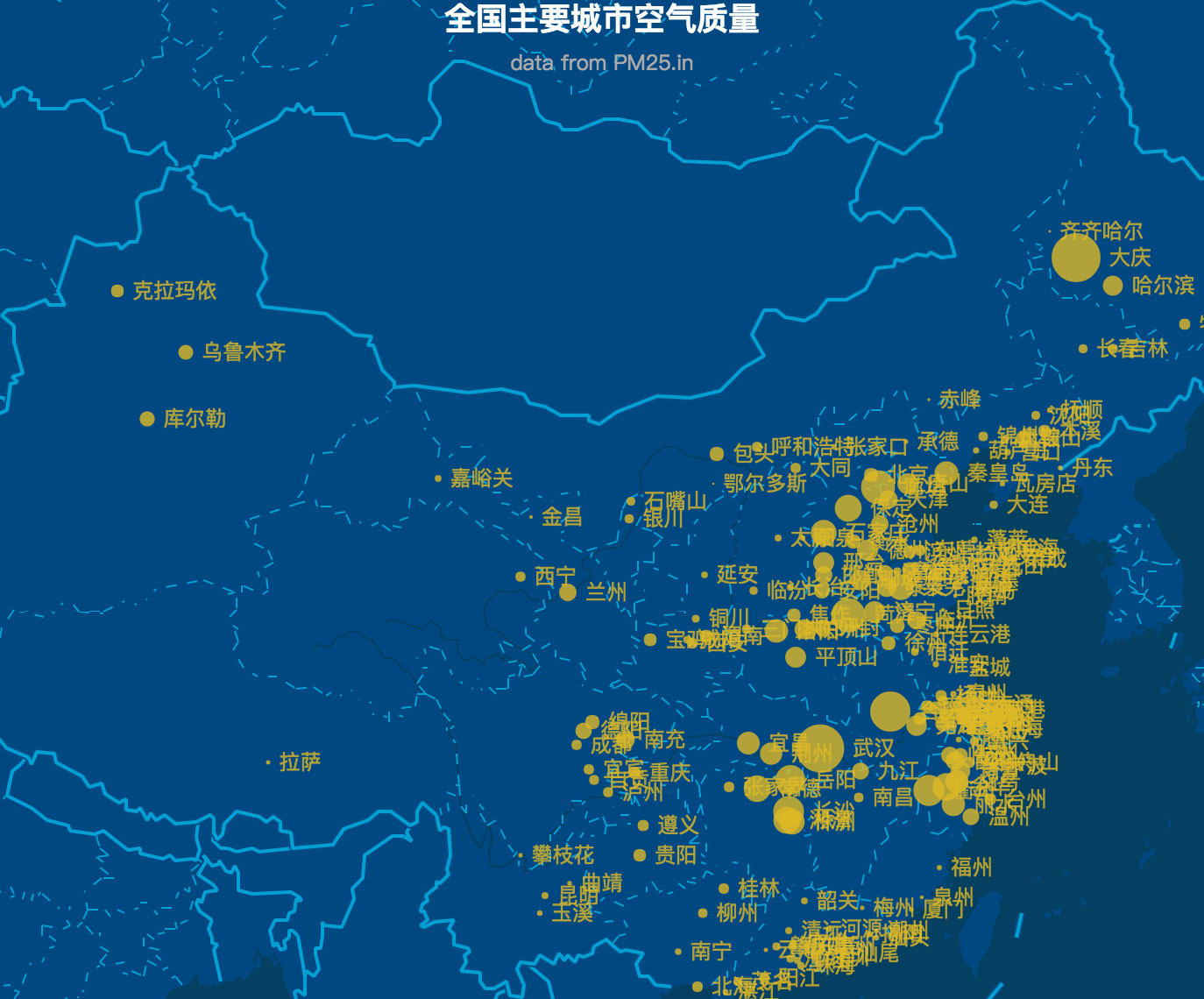inMap 是一款基于 canvas 的大数据可视化库，专注于大数据方向点线面的可视化效果展示。目前支持散点、围栏、热力、网格、聚合等方式；致力于让大数据可视化变得简单易用。

GitHub 地址：https://github.com/TalkingData/inmapinMap 避让算法采用的是四分位模型算法，接下来手把手教你写避让算法，老司机带你装逼带你飞。

## 准备数据

inMap 接收的是经纬度数据，需要把它映射到 canvas 的像素坐标，这就用到了墨卡托转换，墨卡托算法很复杂，以后我们会有单独的一篇文章来讲讲他的原理。经过转换，你得到的数据应该是这样的：

``````[
{
"name": "海门",//要显示的文字
"lng": 121.15,
"lat": 31.89,
"count": 7,
"pixel": { //像素坐标
"x": 968,
"y": 736
}
},
{
"name": "鄂尔多斯",
"lng": 109.781327,
"lat": 39.608266,
"count": 5,
"pixel": {
"x": 659,
"y": 478
}
},
...
]
``````

## 求出每段文字矩形的实际大小

`measureText()` 是 canvas 内置的方法，返回字体宽度的像素单位：

``````let ctx = this.container.getContext('2d'); // canvas 上下文
let width= ctx.measureText(name).width;
``````

``````let fontSize = parseInt(ctx.font);
let height = fontSize * 1.1;
``````

## 创建四分位模型``````_getLeftAnchor() {
let x = this.center.x - this.radius - this.textReact.width,
y = this.center.y - this.textReact.height / 2,
maxH = diam > this.textReact.height ? diam : this.textReact.height; //矩形的高度
return {
x,
y,
minX: x,
minY: this.center.y - maxH / 2,
maxY: this.center.y + maxH / 2
};
}
``````

## 判断碰撞

``````/**
* 判断分位是否相交
* @param {*} target
*/

isAnchorMeet(target) {
let react = this.getCurrentRect(),
targetReact = target.getCurrentRect();
if ((react.minX < targetReact.maxX) && (targetReact.minX < react.maxX) &&
(react.minY < targetReact.maxY) && (targetReact.minY < react.maxY)) {
return true;
}
return false;
}
``````

## 创建虚拟文字集合对象

``````let labels = pixels.map((val) => {
return new Label(val.pixel.x, val.pixel.y, radius, fontSize, byteWidth, val.name);
});
``````

``````do {
var meet = false; //本轮是否有相交
for (let i = 0; i < labels.length; i++) {
let temp = labels[i];
for (let j = 0; j < labels.length; j++) {
if (i != j && temp.show && temp.isAnchorMeet(labels[j])) {
temp.next();
meet = true;
break;
}
}
}
} while (meet);
``````

## 绘画文字

``````labels.forEach(function (item) {
if (item.show) { //是否显示
let pixel = item.getCurrentRect();
ctx.beginPath();
ctx.fillText(item.text, pixel.x, pixel.y);
ctx.fill();
}
});
``````

1 回复